# Exploring the Calculation Dialog Box

Now that you know something about how and where calculation formulas are used, it's time to turn next to the layout of the calculation dialog box itself. There are some small differences among the calculation dialogs you find in particular areas within FileMaker Pro. We'll focus our attention on the dialog used for defining calculation fields because it's the most complex. Figure 8.3 shows the calculation dialog for a field called FullName, which serves as the model for this anatomy lesson.

Figure 8.3. When you're creating calculation fields, it helps to know your way around the Specify Calculation dialog box.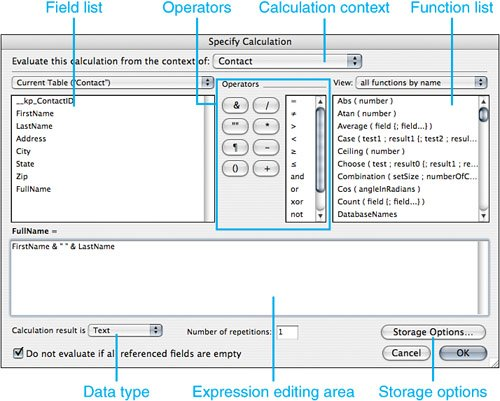Specifying Context

Across the top of the dialog, you're asked to specify the context from which to evaluate this calculation. This choice is necessary only when the source table you are working with appears in your Relationships Graph more than once. And even in those cases, it really matters only when your calculation formula involves related fields. In such cases, the calculation may return different results, depending on the context from which it's evaluated.

To make this point clear, consider the example in Figure 8.4.

Figure 8.4. Notice that the Transaction table occurrence has two related parent table occurrences derived from the same source table of Person.In this example, a person can act as either a buyer or a seller for a given transaction. This means then that a person record will have potentially two sets of related transactions: those for which that person is a seller, and those for which he or she is a buyer. If you were to create a calculation field in the Person table that produced a total of transactions, you'd get very different results depending on from which context you calculate. Both versions of the calculation would use a SUM ( Transaction::Amount ) formula, but the originating context will determine whether you're viewing records from the buyer perspective or the seller perspective. In this example, it's critical that the originating context be set deliberately in order to determine which transactions get totaled.

Writing the Formula

The large box in the middle of the Specify Calculation dialog is where you define the formula itself. If you know the syntax of the functions you need and the names of the fields, you can simply go ahead and type in the formula by hand. In most cases, though, you'll want to use the lists of fields and functions above the text box. Double-clicking on an item in those lists inserts that item into your formula at the current insertion point.

Note

On a Macintosh, after an item is highlighted, you can also press the spacebar to insert it into your formula. On Windows, the Insert key functions similarly.

Every calculation formula is made up of some combination of fields, constants, operators, and functions. All the following are examples of formulas you might write:

```2 + 2
FirstName & " " & LastName
Get(CurrentDate) + 14
Left( FirstName; 1 ) & Left ( LastName; 1 )
"Dear " & FirstName & ":"
\$loopCounter = \$loopCounter + 1
LastName = "Jones"```

In these examples, FirstName and LastName are fields. \$loopCounter is a variable by virtue of being prefixed with a dollar-sign character. Get ( CurrentDate ) and Left are functions. The only operators used here are the addition operator (+) and the concatenation operator (&). (Concatenation means combining two text strings to form a new text string.) There are also numbers and text strings used as constants (meaning that they don't change), such as 14, "Dear", and "Jones". Text strings are the only things that need to be placed within quotes. FileMaker assumes that any unquoted text in a formula is a number, a function name, or a field name. If it's none of these, you get an error message when you attempt to exit the dialog.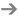To learn about variables, see Chapter 15, "Advanced Scripting Techniques," p. 435.

Selecting Fields

In the calculation dialog, above the formula box to the left is a list of fields. By default, the fields in the current table are listed. You can see the fields in a related (or unrelated) table by making a selection in the pop-up above the field list. Double-click a field name to insert it into your formula. You can also type field names directly.

Caution

Be aware that the only fields you can use from an unrelated table are those with global storage. There's no way FileMaker could determine which record(s) to reference for nonglobally stored fields. You get an error message if you attempt to use a nonglobal field from an unrelated table in a formula.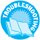If you're having difficulty with field name syntax in formulas within ScriptMaker, see "Formulas in Scripts Require Explicit Table Context" in the "Troubleshooting" section at the end of this chapter.

Choosing Operators

In between the field and function areas in the Specify Calculation dialog is a list of operators you can use in your formulas. Operators are symbols that define functions, including the math functions addition, subtraction, raising to a power, and so on.

Note

Strictly speaking, not all the symbols listed here are operators. The ¶ paragraph symbol (or pilcrow), for instance, is used to represent a literal return character in strings.

There is often some confusion about the use of &, +, and the and operator. The ampersand symbol (&) is used to concatenate strings of text together, as in the previous example in which we derive the FullName by stringing together the FirstName, a space, and the LastName. The + symbol is a mathematical operator, used, as you might expect, to add numbers together. The and operator is a logical operator used when you need to test for multiple Boolean conditions. For instance, you might use the formula Case (Amount Due > 0 and Days Overdue > 30, "Overdue"). Here, the and indicates that both conditions must be satisfied for the test to return true.

The other operators are quite intuitive, with the exception of xor. xor, which stands for exclusive or, is used to test whether either of two statements is true, but not both of them. That is, (A xor B) is the same thing as "(A or B) and not (A and B)." The need for such logic doesn't come up often, but it's still handy to know.

Selecting Functions

The upper-right portion of the Specify Calculation dialog contains a list of the functions you can use in your formulas. By default, they are listed alphabetically, but you can use the View pop-up menu above the list to view only formulas of a certain type. The Get functions and External functions, in fact, will display only if you change to View by Type.

Double-clicking a function inserts the function into your formula at the current insertion point. Pressing the spacebar (Macintosh) or the Insert key (Windows) while the function is highlighted also adds the function to your formula. The "guts" of the functionthe portion in between the parenthesesis highlighted so that you can begin typing parameters immediately.To learn more about how to read and use functions, see "The Parts of a Function," p. 229.

Writing Legible Formulas

Whether you're typing in a formula by hand or are using the selection lists to insert fields and functions, we have a few general comments about how to make your functions easy to read.

First of all, when you're writing functions, spacing, tabs, and line returns don't matter at all. You can put spaces, tabs, and returns just about anyplace you want without changing how the formula evaluates. For legibility, it's therefore often helpful to put the parameters of a function on separate lines, especially when you have nested functions.

You can also add comments to calculation formulas. You can prefix a comment with two forward slashes (//) and anything following on that line will not be evaluated. To comment a block of multiple-lined text, begin with /* and close with */.

Compare, for example, the legibility of a complex function written two different ways. In Figure 8.5, you can see a mildly complex function with no commenting or spacing. In Figure 8.6, that same formula has been rewritten with comments and extra spacing to make it more legible. Legibility isn't merely an idle concern; it has real value. If you, or someone else, ever need to debug or alter one of your formulas, it will take much less time and effort if you've formatted your formula well in the first place.

Figure 8.5. A complex formula written without adequate spacing can be very difficult to understand and troubleshoot.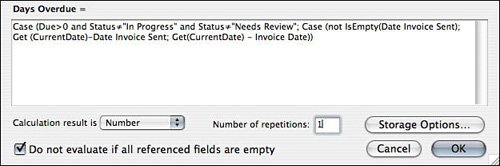Figure 8.6. Adding spaces, returns, and comments to a formula can make it much more legible, and hence easier to maintain in the future.Other Options

Before ending this calculation dialog anatomy lesson, we must cover the miscellaneous options you can see at the bottom of the Specify Calculation dialog. These options pertain only to defining calculation fields; you don't see them in any of the other calculation dialogs.

Data Type

The first of these miscellaneous options is to specify the type of data the calculation will return. Usually, it's quite obvious. If you're concatenating the FirstName and LastName fields to form the FullName field, your calculation result will need to be a text string. If you're adding the SalesTax to an InvoiceSubTotal to generate the InvoiceTotal, the expected result will obviously be a number. Adding 14 days to the current date to generate a callback date should result in a date. Simply ask yourself what type of data the formula should produce and select the appropriate result.If you do choose the wrong data type for a calculation field, you may experience some unexpected results. See "Errors Due to Improper Data Type Selection" in the "Troubleshooting" section at the end of this chapter.

Number of Repetitions

The only time you'll ever have to worry about the number of repetitions in a calculation field is when your formula references one or more repeating fields. If it does, you'll typically define your calculation to have the same number of repetitions as the fields it references. The formula you define is applied to each repetition of the source fields, resulting in different values for each repetition of your calculation field.

If you reference nonrepeating fields in your calculation, they affect only the first repetition of output. You can, however, use the Extend() function to allow a nonrepeating field to be applied to each repetition of output.

For instance, in Figure 8.7, Quantity and Line Cost are both number fields defined to allow 10 repetitions. Tax Rate is a regular number field. The formula used to determine the LineTotal is as follows:

`Quantity * LineCost * (Extend(TaxRate) + 1)`

Figure 8.7. Calculation fields can be defined to allow multiple repetitions.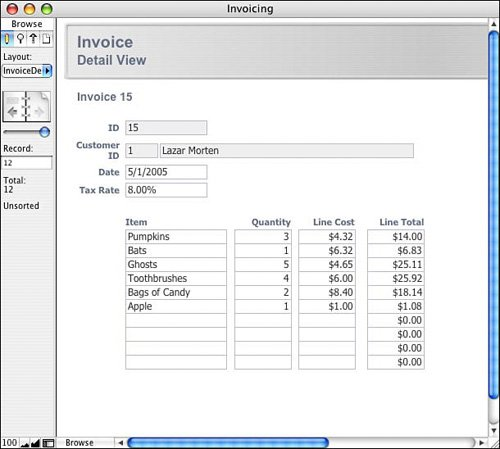LineTotal itself is defined to allow 10 repetitions.

Do Not Evaluate

By default for new calculation fields, the Do Not Evaluate If All Referenced Fields Are Empty box on the Specify Calculation dialog is checked. This means that the calculation will return a null (empty) value as long as all the fields it refers to are empty. If this box is unchecked, the formula will be evaluated using the empty values in the referenced fields. For instance, say you had a StatusCode field in an invoice database and wanted to use it to generate a status message, the formula of which was If ( StatusCode = "P"; "Paid"; "Not Paid" ). If you left the Do Not Evaluate... box checked, invoices with no status code would have no status message. If it were unchecked, their status message would be Not Paid.

Another example draws from this feature's most common use: financial calculations. If you have a field that calculates, say, a price total based on quantity and sales tax fields, it's often helpful to return an explicit zero rather than leaving the calculation field null or blank.

Consider a calculation field that calculates a discount based on a transactionAmount field:

`If ( transactionAmount >= 1000; 50; 0 )`

If the check box is unchecked, this evaluation will return a zero if either transactionAmount is less than 1000 or the field is empty. In this way, the zero is explicit and demonstrates for the user that the calculation was performed. If the check box is left checked and transactionAmount is empty, this discount field will be empty as well, leading to possible ambiguity on the part of users.

There's no simple rule we can provide as to when you want to check or uncheck this option. You need to look at your formula and determine whether the inputs to the formula (those fields referenced in the formula) could all ever be blank, and if so, whether you would still want the formula to evaluate. Typically, if your formulas have default results (as in the StatusCode example) rather than using explicit logic for determining results, you probably want to uncheck the box.

Storage Options

The last things we'll touch on in this anatomy lesson are the storage options available when you're defining calculation fields. Be aware that the output of your calculation formula may differ depending on the storage method selected. The Storage Options dialog box is shown in Figure 8.8.

Figure 8.8. The Storage Options dialog enables you to set calculation fields so that they have global results and to specify indexing options.In the top portion of the dialog, you may specify global storage as an option. This is a concept introduced in FileMaker Pro 7, and one perhaps not immediately intuitive even for longtime FileMaker developers. Global storage for regular fields (that is, text, number, date, time, timestamp, or container) is typically used when you need a temporary storage location for a value or for infrequently changing, solutionwide values such as your company's name and address. For instance, globally stored text fields are often used in scripts as a place to hold users' preferences or selections as they navigate through your interface.For more information on global storage of field data, see "Storage and Indexing," p. 86.

If you set a calculation field to be stored globally, the results of the calculation formula will be available to you from any record, and indeed, any table, in your system without having to establish a relationship to a table occurrence tied to its source table. The formula isn't evaluated for each record in the system; it is evaluated only when one of the inputs of the formula changes or when you modify the formula.

Consider a scenario involving a sales commission calculation. You might create a utility table containing the fields necessary to calculate a daily sales commission (based on market values or whatever variable data affected the business in question) in which a manager could modify the data in the formula on demand. A global calculation then would provide the system with its current sales commission without requiring a series of relationships.

Note that this example assumes there to be one record in the utility table in question. If there were multiple records, it would be possible to include the concept of an active/inactive status into the calculation or simply rely on the fact that the last edited record will be that from which the calculation will draw its source information.

The bottom half of the Storage Options dialog enables you to specify indexing options. Indexing a field speeds up searches based on that field, but it results in larger files. FileMaker also uses field indexes for joining related tables.For more detailed information on indexing, see "Storage and Indexing," p. 86.

In most cases, the default indexing option for a calculation field will be set to None, and the Automatically Create Indexes as Needed box will be checked. For most calculations you write, this configuration is perfect. FileMaker determines whether an index is needed and creates one if it is. Performing a find in the field or using the field in a relationship are both actions that trigger the automatic indexing of a field.

For some calculation formulas, the default storage option is to have the Do Not Store Calculation Results option checked and for everything else to be grayed out. This is an indication that the field is unindexable. Calculation fields that return text, number, date, time, or timestamp results can be indexed as long as they are stored. Calculations can be stored as long as they don't reference any unstored calculations, globally stored fields, related fields, or summary fields.

There are a few circumstances in which you'll want to explicitly turn off storage. For instance, when you use any of the Get functions in a calculation, you should make sure that the calculation result is unstored. (Get functions typically return information relating to the state of one's user session. By definition, that information changes on a second-by-second basis, and formulas based on it should not be stored so that they continue to reflect present reality.) If you do so, the calculation is forced to evaluate based on the current environment each time it's evaluated (as opposed to always "remembering" the environment at the time the record was created or modified). Imagine you defined a calculation to return the number of records in the current found set by using the Get ( FoundCount ) formula. If you don't explicitly set the results to be unstored, then for a given record, the formula evaluates once and keeps that value, regardless of changes to the size of the found set. The count of found records the first time the calculation is triggered is the value that will be stored. As their name implies, unstored calculations do not make your files larger, but because they must evaluate each time you view them, they can slow down a system if they're based on complex formulas.

As a rule of thumb, you should stick with the default storage options unless you know for sure that you need the result to be unstored. You'll almost never need to explicitly turn indexing on; let FileMaker turn it on as necessary. Very seldom should you uncheck the option to have FileMaker turn on indexing as needed. Be aware that indexing increases the size of your files, sometimes by a great deal. By unchecking the option to have FileMaker turn on indexing as needed, you can ensure that certain fields won't be indexed accidentally just because a user performs a find on them.

### Essential Functions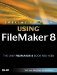Special Edition Using FileMaker 8
ISBN: 0789735121
EAN: 2147483647
Year: 2007
Pages: 296